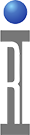The Deltas 1-input calc button can be used to easily compute the differences between steps in an array. For example, the Differential Non-Linearity (DNL) and Integral Non-Linearity(INL) of a Digital Step Attenuator (DSA) can be computed using a couple of test panels and this calc button. The Detlas' button help says, "Return a new data that is the result of calculating the delta difference between each set of adjacent values. The data returned is one size smaller than the original data (except if the original data was of size 1 to begin with)".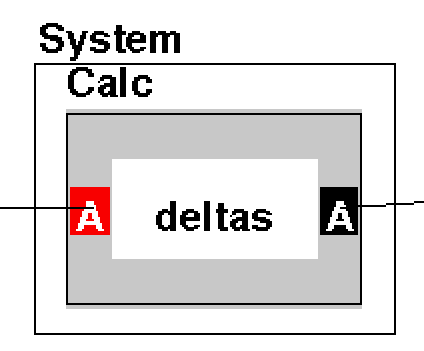For example, a Digital Step Attenuator with 24 steps, the DNL would have 23 values. The formula and table below can be used to illustrate this calculation. This can be accomplished with a few test panels and standard System Calc buttons. In the example panels below, we are using DutControl Voltage measurement. In an actual testplan, you would use the DUT's step control commands as the index used to generate the data. So replace DutControl > Vcc sweep from 1 to 24 in 24 points with a similar sweep using the Dut instrument.

The formula for computing DNL of step "i" is the following: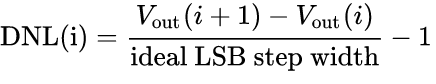Digital Step Attenuator (DSA) steps in an array (sweep values)
Gain is array with measurement value for each step.
Delta Gain is difference between each step. (Numerator in the equation above)
DNL is "Delta Gain-1" (/1 being the ideal LSB step width)
Expected is -1 db each step from start.
INL is "Gain - Expected" which is how far off from ideal the device is performing

Table 1: Example DSA Calculation
 DSA Steps Gain Delta Gain DNL Expected INL 1 13.719751613.7197516 0 2 12.7983552 -0.9213964 -1.9214 12.7197516 -0.0786 3 11.879749 -0.9186062 -1.91861 11.7197516 -0.16 4 10.9941127 -0.8856363 -1.88564 10.7197516 -0.27436 5 10.1447348 -0.8493779 -1.84938 9.7197516 -0.42498 6 9.22399144 -0.92074336 -1.92074 8.7197516 -0.50424 7 8.30873731 -0.91525413 -1.91525 7.7197516 -0.58899 8 7.42384829 -0.88488902 -1.88489 6.7197516 -0.7041 9 6.57202686 -0.85182143 -1.85182 5.7197516 -0.85228 10 5.65942136 -0.9126055 -1.91261 4.7197516 -0.93967 11 4.75682626 -0.9025951 -1.9026 3.7197516 -1.03707 12 3.8741662 -0.88266006 -1.88266 2.7197516 -1.15441 13 3.00424819 -0.86991801 -1.86992 1.7197516 -1.2845 14 2.1011013 -0.90314689 -1.90315 0.7197516 -1.38135 15 1.18932807 -0.91177323 -1.91177 -0.2802484 -1.46958 16 0.317622547 -0.871705523 -1.87171 -1.2802484 -1.59787 17 -0.543283335 -0.860905882 -1.86091 -2.2802484 -1.73697 18 -1.45680009 -0.913516755 -1.91352 -3.2802484 -1.82345 19 -2.35144422 -0.89464413 -1.89464 -4.2802484 -1.9288 20 -3.22966889 -0.87822467 -1.87822 -5.2802484 -2.05058 21 -4.10045402 -0.87078513 -1.87079 -6.2802484 -2.17979 22 -4.97876011 -0.87830609 -1.87831 -7.2802484 -2.30149 23 -5.90239336 -0.92363325 -1.92363 -8.2802484 -2.37786 24 -6.75234653 -0.84995317 -1.84995 -9.2802484 -2.5279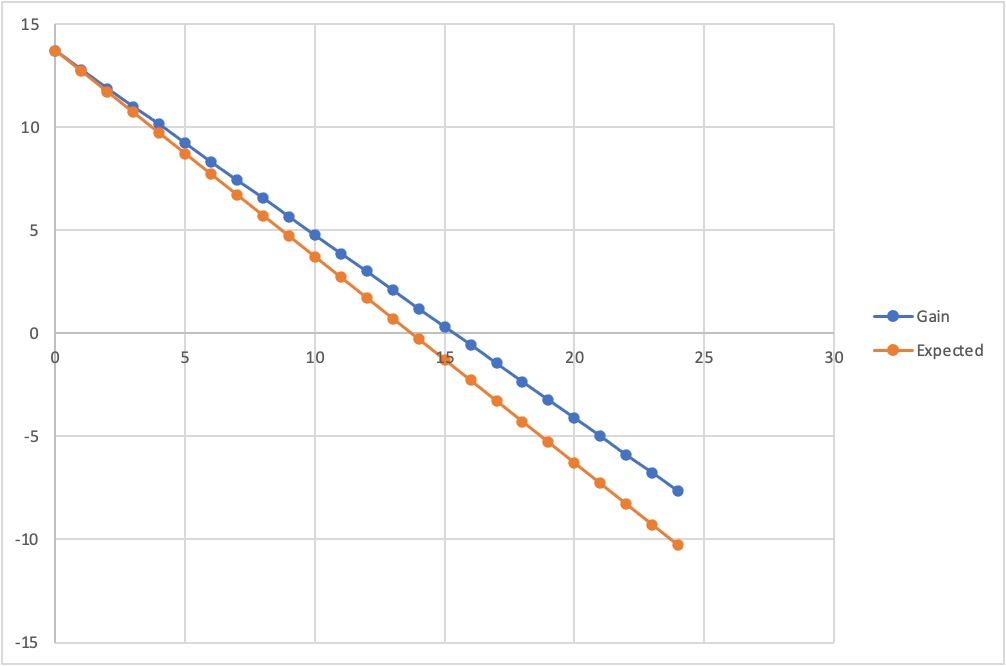Sample Testplan: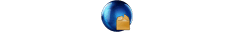DSA_DNL_INL_Example_RiTestplan.gzp (Use Guru Browser to import this testplan with annotated Test NOTES titled "DSA_DNL_INL")

To Use deltas Calc button to Compute DNL:
1. Use the Device Control editor to create a button which can control the DUT into it's various steps that align with the start, stop and points values of a sweep with the Dut instrument. This becomes the "index" of the array.
2. From the Cassini Testplan Editor, choose Edit > Add Test Section, rename it to "Sweep" and then Edit > Add Test and name it "DSA Sweep". Use Tester > View and copy buttons needed to create a test which connects the Receiver's measurement output to a System > Array button to store the Gain data, name it "DSA_Sweep". The example also saves the results to the datalog for additional validation, this is not required for the calculation. (See Figure 1)
3. Add another Test which calculates the expected gain values for the sweep. In this example we have 1 dB steps so the expected value is always -1 dB down from the first value. In an actual testplan, replace the Vcc sweep with the Dut control sweep and the DUT > Inst State Source value would still be correct. (i.e. the count of the step) (See Figure 2)
4. Add another Test which calculates the gain deltas. Reduce the sweep size by 1 and connect the gain array to a 1 input calc button. Choose Calc... from the button's right mouse button menu and choose deltas.(See Figure 3)
5. Connect the System Calc deltas ouput to a System Array called DSA_Deltas. (See Figure 4)
6. Add another Test which calculates the DNL by subtracting 1 from the Deltas. (note: Deltas and DNL test panels could be easily combined.)
7. Add a final Test which calculates the INL by calculating the difference between measured and ideal performance. (See Figure 5)
8.

Figure 1: Digital Step Attenuator Sweep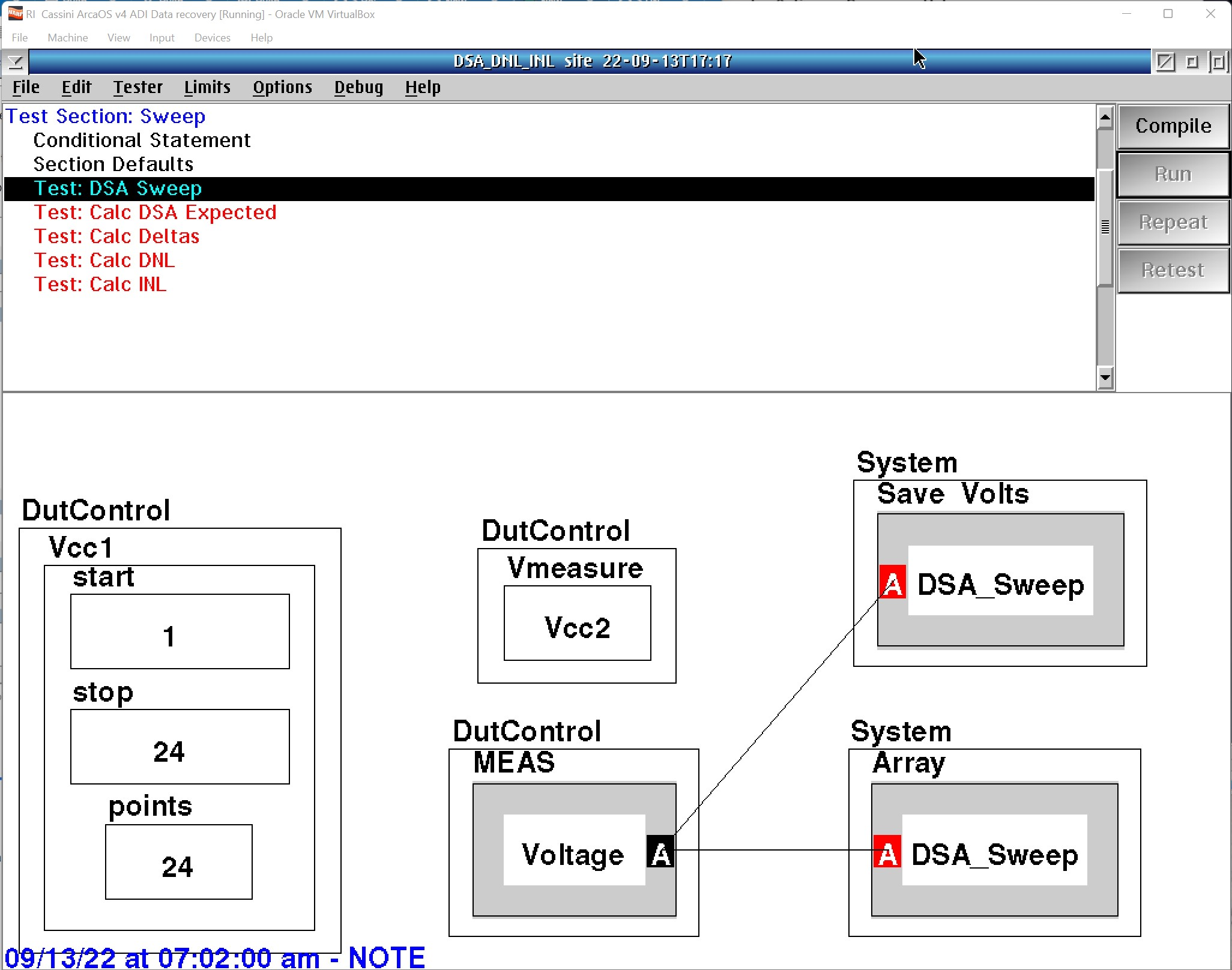Figure 2: Calc DSA Expected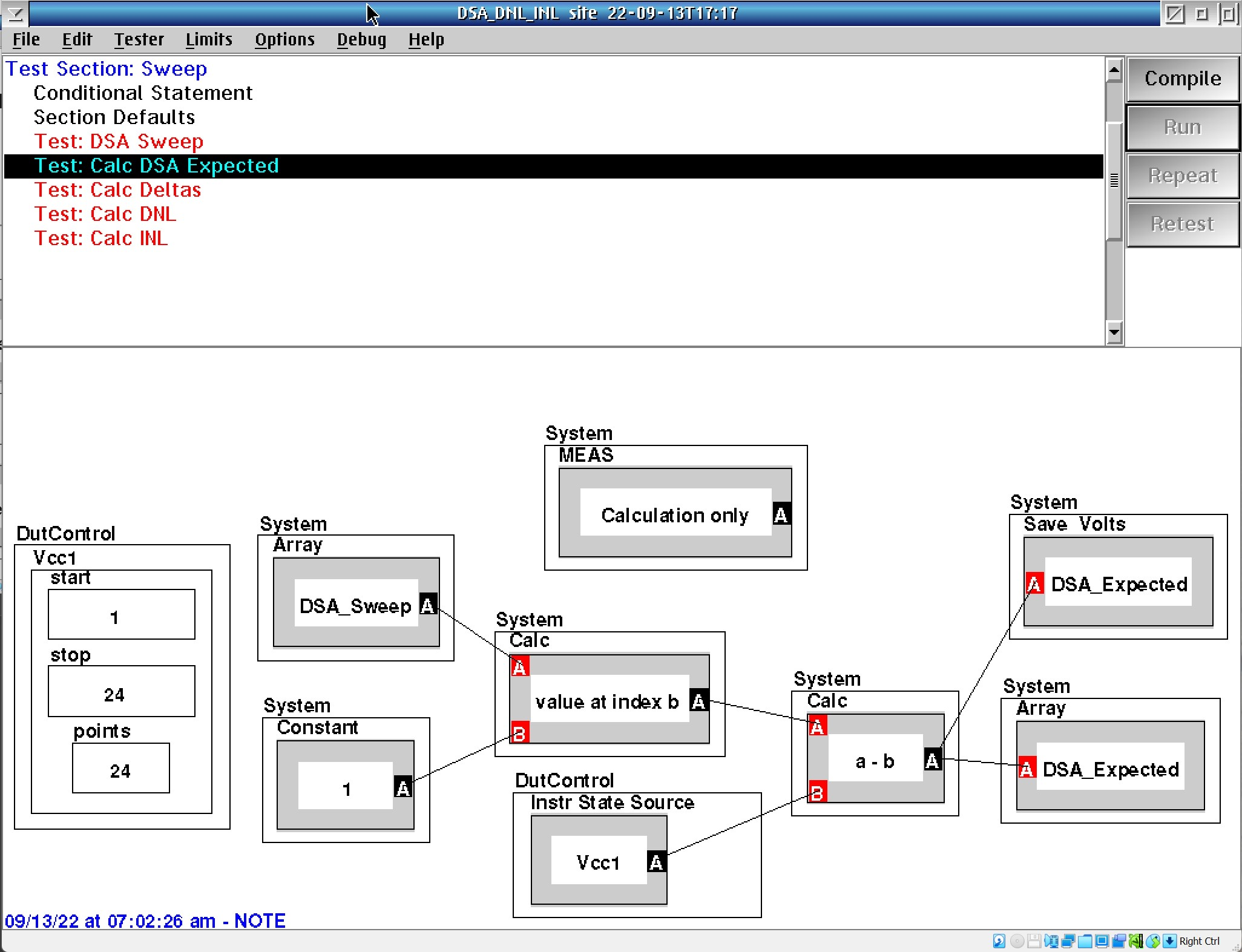Figure 3: Calc > Gain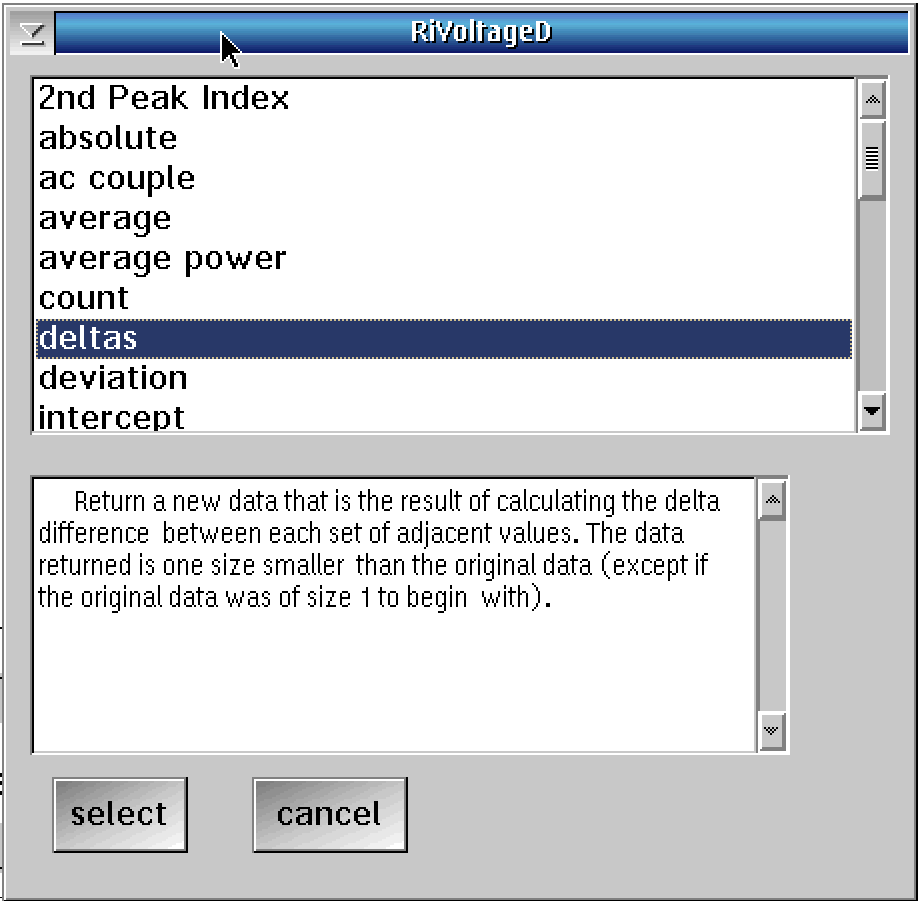Figure 4: Calc Gain Deltas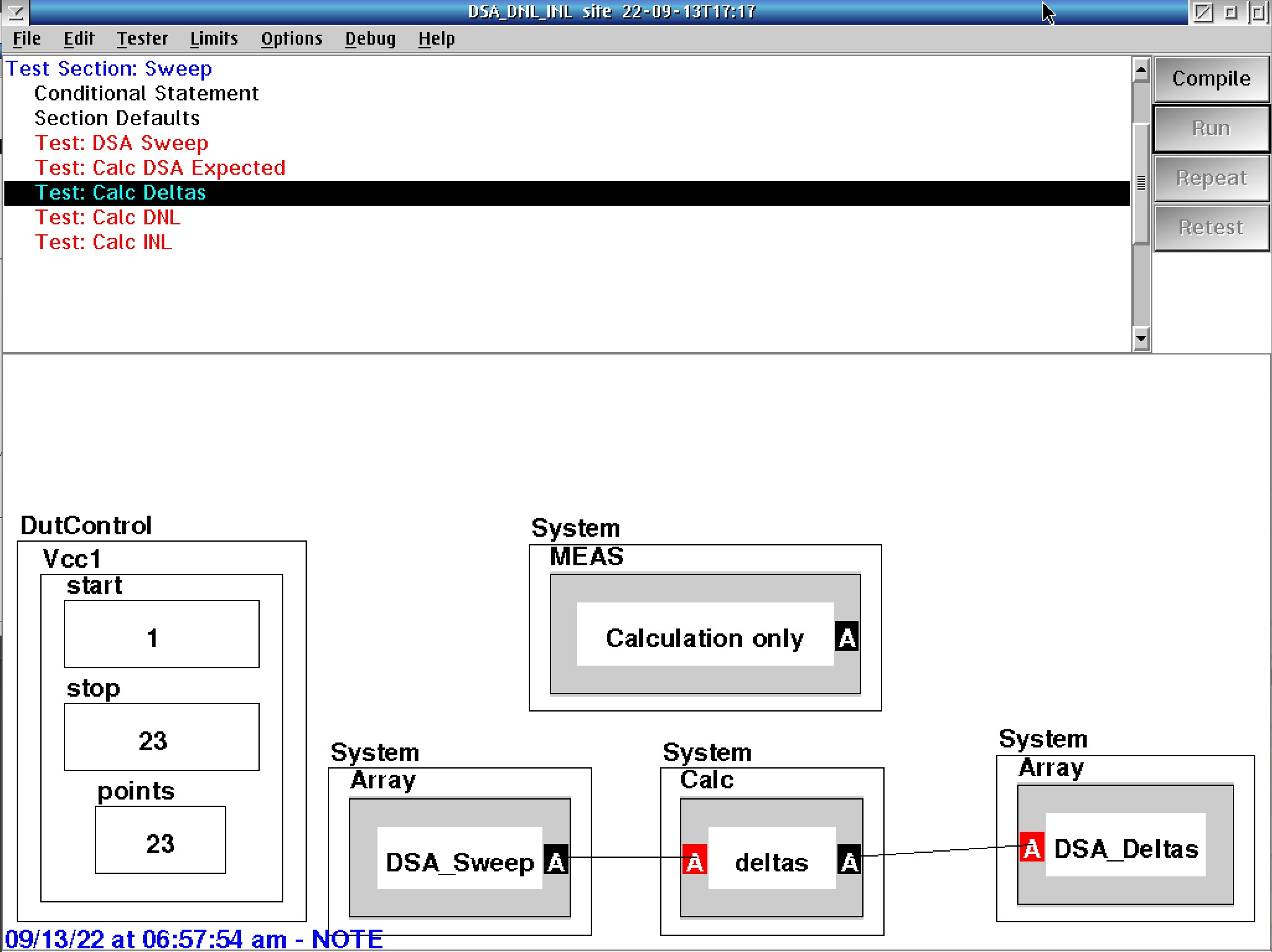Figure 5: Calc DNL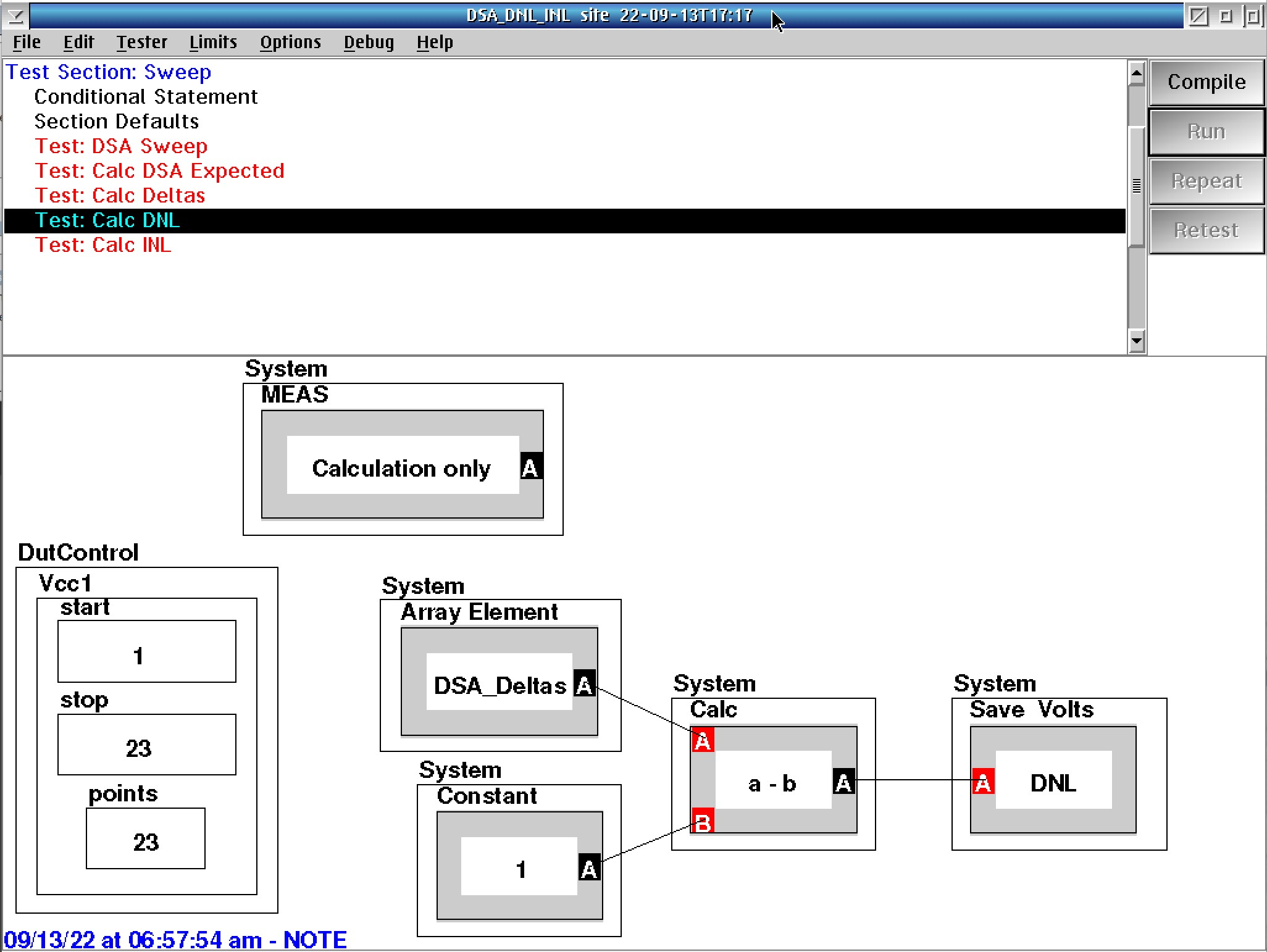Figure 6: Calc INL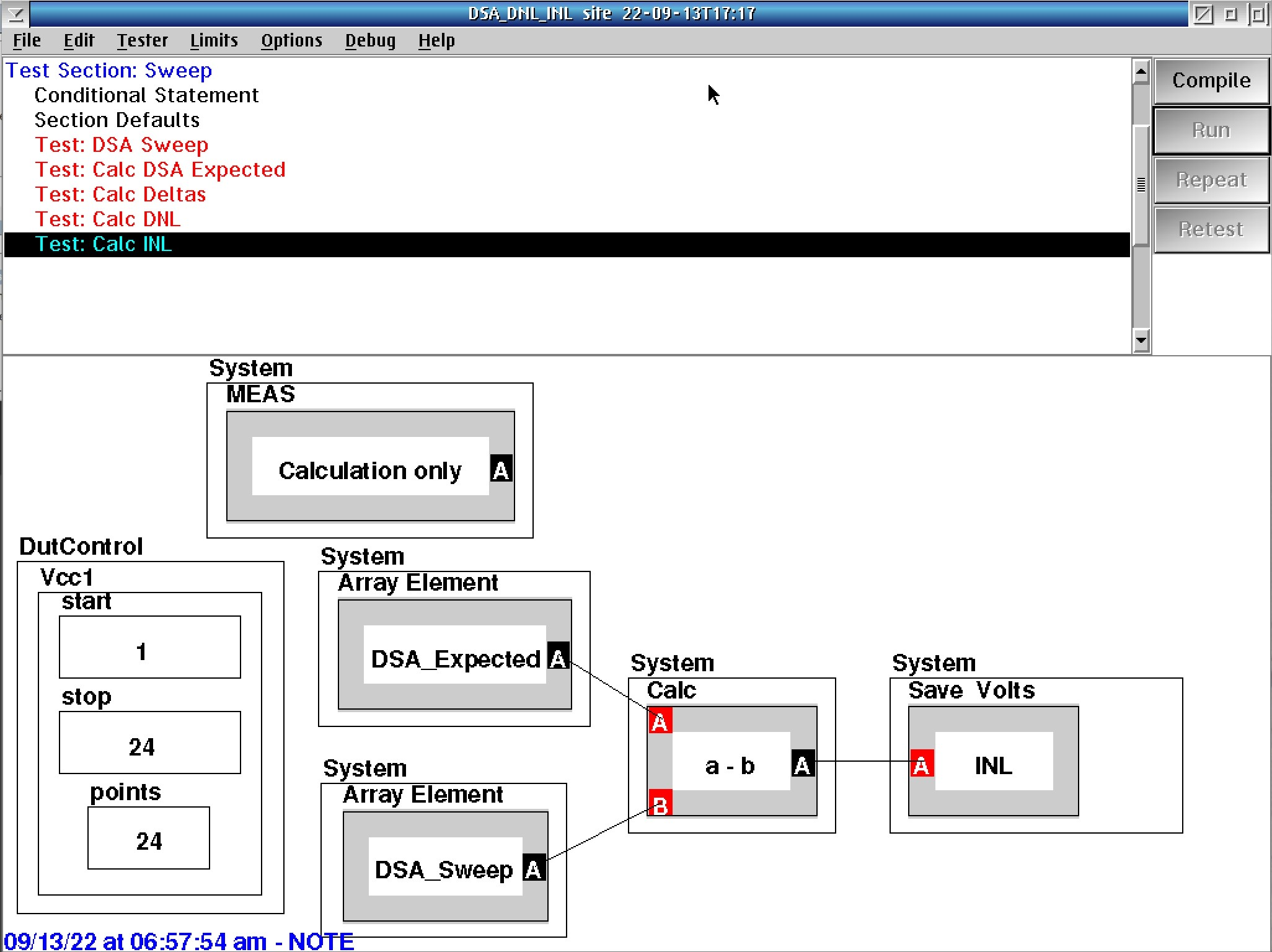https://roos.com/docs/RBEH-CJ8KKV
ROOS INSTRUMENTS CONFIDENTIAL AND PROPRIETARY